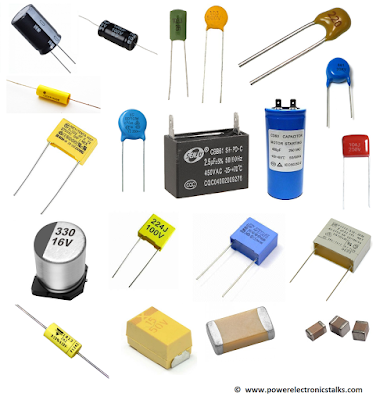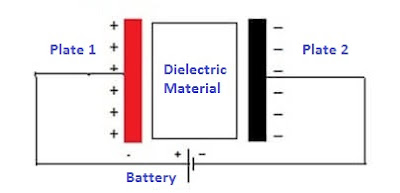# Capacitor

Capacitors are most frequently used component in electronic circuits. Whatever types and values a selection of correct capacitor solves many circuit issues. In this article we will discuss definition of capacitor, capacitor symbol, principle of capacitor, capacitor unit and capacitor working.

# What is a Capacitor?

Capacitors are electronic components which can momentarily store electrical charge, and whose working is determined by how much electric charge can be stored in it.Different Types of Capacitors

A capacitor is an electronic component having two terminals. Capacitor is a passive component. Capacitor stores electric energy. Capacitance we can define as the effect of a capacitor. Sometimes it is called as a condenser or condensator.

### Capacitance

The effect created by a capacitor is known as capacitance. Also we can define the capacitance as the ratio of the electric charge on each conductor to the potential difference between.

In other words we can say that, the capacitance is defined as the ratio of charge Q on a conductor to a potential difference V between them.

C=Q/V

### Capacitor Unit

The unit of capacitance is the Farad (F) which is the International System of Units (SI). In general electronics, ranges from 1 picofarad (pF) to 1 millifarad (mF) capacitance values are used.

### Capacitor SymbolCapacitor Symbols

First is fixed capacitor i.e. non polarized capacitor and this capacitor can be used in both AC and DC circuit. This capacitor is “bilateral element” i.e. polarity doesn't matter during connection.

Second is varying capacitor. Here capacitance can be vary.

Third is polarized capacitor. During its connection here we have to take care of its polarity. This capacitor is use in a DC circuit.

### Principal of Capacitor

Below points will explain the principal of capacitor in detail;

• Most of the capacitors have at least two electrical conductors’ i.e. metallic plates, in other words we can say that outsides parted or separated by a dielectric medium.
• These conductors are may be a thin film, a foil, sintered bead of metal or a part of electrolyte.
• The capacitor's charge capacity is increase by the non-conducting dielectric material.
• Dielectric materials in capacitors are commonly used as paper, plastic film, glass, mica, ceramic, cellulose, porcelain, Mylar, Teflon and layers of oxide. Sometimes air also used as non-conductive medium.
• When a potential difference like DC voltage from battery is applied across the two conductors, an electric field grows across the dielectric material, producing a positive charge to gather on one plate and negative charge to gather on the other plate of capacitor.Dielectric in ceramic disc capacitor
• Dielectric never allows current to flow across it. Though, there is a flow of charge through the applied source circuit. If this state is continued adequately for long, the current through the applied source circuit ends.
• If a varying voltage is applied across the two leads of the capacitor, the applied source undergoes an on-going current because of the charging and discharging cycles of the capacitor.
• Capacitor didn’t dissipate power in the form of heat.

### Capacitor working

Below points will explain the working of capacitor in detail;

• The working of capacitor based on Coulomb’s law. A charge on one conductor employs a force on the charge carriers in the other conductor, enticing opposite polarity charge and deterring like polarity charges, thus an opposite polarity charge will be made or induced on the surface of the other conductor.
• When supply is applied from a battery or a power supply, there is flow of current i.e. flow of electron will occur. But there is a dielectric medium in capacitor which stops the flow of current between the plates. So these electrons will start gathering on the plates. Up to a definite level, the charge can be deposited or stored. This shows that the capacitor is charged fully.
• The two plates in capacitor have an equal amount of positive and negative charges producing a strong electric field which makes them to hold this charge inside the capacitor.
• If the capacitor is fully charged and if the load is connected to a capacitor, it will supply energy to load until it converts to electrically neutral.
Let understand working of capacitor with the help of below figure;Capacitor working
• A battery (DC Source) is connected across a capacitor i.e. one surface of the capacitor which is named as plate 1 gets positive end of the battery and another surface i.e. second surface of the capacitor which is named as plate 2 gets negative end of the battery.
• With the application of battery or DC voltage, the full voltage of the battery is applied across the capacitor. At this condition, plate 1 of capacitor is in positive energy with respect to the plate 2.
• Current from the battery or DC supply source tries to flow through the capacitor from its positive plate (plate 1) to negative plate (plate 2) but cannot flow with large amount because these plates are separated with a dielectric insulating material.
• Somewhat a very small current will flow through this dielectric insulating material from Positive side plate to Negative side plate, which depends on the value of strength of this dielectric material.
• Now an electric field will generate or form inside the capacitor i.e. inside the dielectric from positive plate to negative plate.
• As the time passes, the positive plate (i.e. plate 1) will gather positive charge from the battery or DC source and negative plate (i.e. plate 2) will gather negative charge from negative side of the battery or power source.
• After a certain time, the capacitor carries maximum quantity of charge according to its capacitance with respect to the applied voltage. This time duration is called as charging time of the capacitor.
• If two sides of capacitor (i.e. plate 1 and plate 2) get shorted through a load i.e. a load is connected across the plates, a current will flow across the load from plate 1 to plate 2 until all the charges get disappeared from both the plates. This time duration is called as the discharging time of the capacitor.Electrolytic Capacitors assembled in a circuit

Below are some of the advantages of a capacitor;

• Capacitor stores energy directly into the plates, which results in faster charging and discharging process.

• Capacitors can filter unwanted frequencies very easily.

• Capacitors can be use in power factor correction to handle power loss efficiently and to make economical power generation.

• High and low temperature can’t affect working of a capacitor.

• Capacitors can  discharge current instantly.

• For AC applications Capacitors can be directly use.

• Capacitors are suitable for high frequency applications as they can handle high applied voltages.

• Life span of capacitors are very high. Approximately 15 years for general applications. Military grade capacitors have life more then 15 years.

### Conclusion

Capacitors are most frequently used component of the electronic circuits. Nearly all types of circuits have its presence. There are many types of capacitors and mostly used in electronic circuits for blocking DC while allowing AC to pass and also used for energy storage.